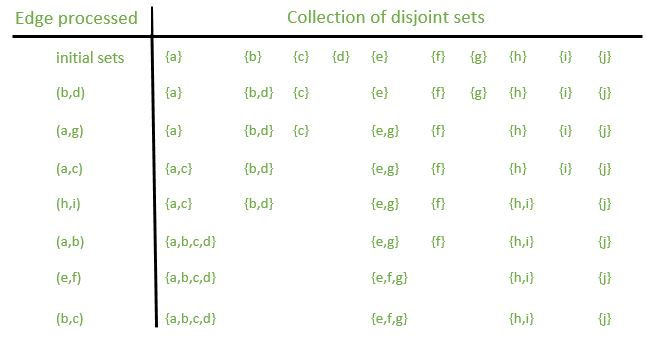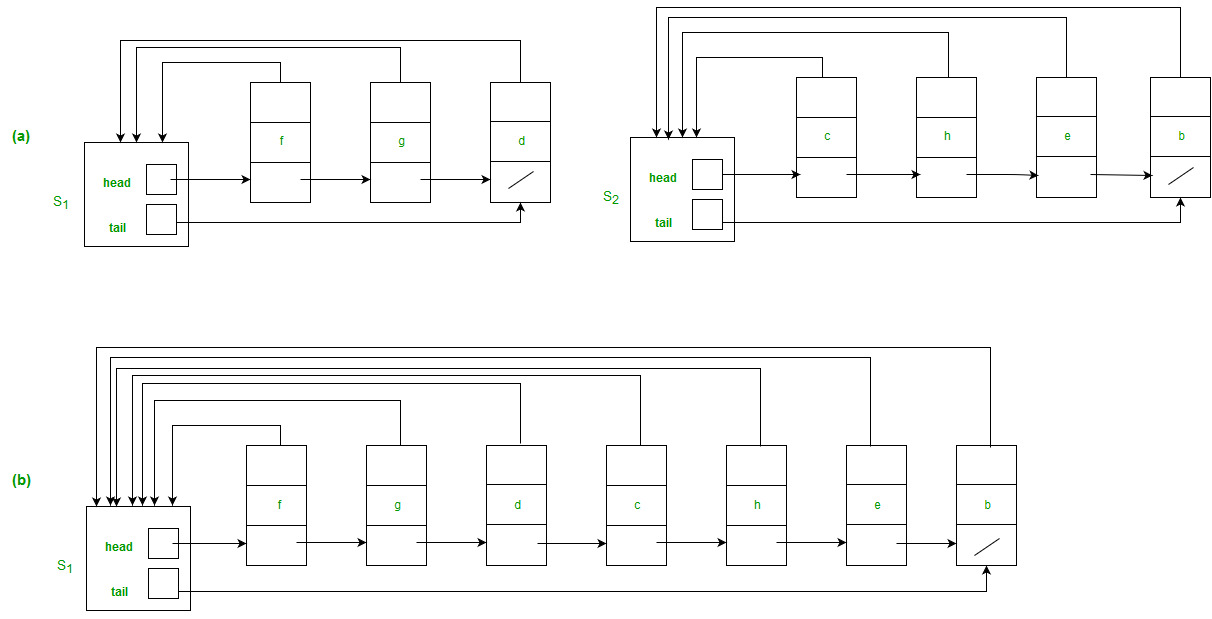Related Articles

# Linked List representation of Disjoint Set Data Structures

• Difficulty Level : Medium
• Last Updated : 14 Aug, 2019

A disjoint-set data structure maintains a collection S = {S1, S2,…., Sk} of disjoint dynamic sets. We identify each set by a representative, which is some member of the set. In some applications, it doesn’t matter which member is used as the representative; we care only that if we ask for the representative of a dynamic set twice without modifying the set between the requests, we get the same answer both times. Other applications may require a prespecified rule for choosing the representative, such as choosing the smallest member in the set.

Example:
Determining the connected components of an undirected graph. Below figure, shows a graph with four connected components.Solution : One procedure X that follows uses the disjoint-set operations to compute the connected components of a graph. Once X has pre-processed the graph, the procedure Y answers queries about whether two vertices are in the same connected component. Below figure shows the collection of disjoint sets after processing each edge.See here as the above example was discussed earlier.Figure (a) Linked-list representations of two sets. Set S1 contains members d, f, and g, with representative f, and set S2 contains members b, c, e, and h, with representative c. Each object in the list contains a set member, a pointer to the next object in the list, and a pointer back to the set object. Each set object has pointers head and tail to the first and last objects, respectively. (b) The result of UNION(e, g), which appends the linked list containing e to the linked list containing g. The representative of the resulting set is f . The set object for e’s list, S2, is destroyed.

Above three figures are taken from the Cormen(CLRS) book. Above Figure shows a simple way to implement a disjoint-set data structure: each set is represented by its own linked list. The object for each set has attributes head, pointing to the 1st object in the list, and tail, pointing to the last object.
Each object in the list contains a set member, a pointer to the next object in the list, and a pointer back to the set object. Within each linked list, the objects may appear in any order. The representative is the set member in the 1st object in the list.
To carry out MAKE-SET (x), we create a new linked list whose only object is x. For FIND-SET(x), we just follow the pointer from x back to its set object and then return the member in the object that head points to. For example, in the Figure, the call FIND-SET(g) would return f.

Algorithm:

Letting x denote an object, we wish to support the following operations:

MAKE-SET(x) creates a new set whose only member (and thus representative) is x. Since the sets are disjoint, we require that x not already be in some other set.

UNION (x, y) unites the dynamic sets that contain x and y, say Sx and Sy, into a new set that is the union of these two sets. We assume that the two sets are disjoint prior to the operation. The representative of the resulting set is any member of Sx U Sy, although many implementations of UNION specifically choose the representative of either Sx or Sy as the new representative. Since we require the sets in the collection to be disjoint, conceptually we destroy sets Sx and Sy, removing them from the collection S. In practice, we often absorb the elements of one of the sets into the other set.

FIND-SET(x) returns a pointer to the representative of the (unique) set containing x.

Based on the above explanation, below are implementations:

 `// C++ program for implementation of disjoint``// set data structure using linked list``#include ``using` `namespace` `std;`` ` `// to represent linked list which is a set``struct` `Item;`` ` `// to represent Node of linked list. Every``// node has a pointer to representative``struct` `Node``{``    ``int` `val;``    ``Node *next;``    ``Item *itemPtr;``};`` ` `// A list has a pointer to head and tail``struct` `Item``{``    ``Node *hd, *tl;``};`` ` `// To represent union set``class` `ListSet``{``private``:`` ` `    ``// Hash to store addresses of set representatives``    ``// for given values. It is made global for ease of``    ``// implementation. And second part of hash is actually``    ``// address of Nodes. We typecast addresses to long``    ``// before storing them.``    ``unordered_map<``int``, Node *> nodeAddress;`` ` `public``:``    ``void` `makeset(``int` `a);``    ``Item* find(``int` `key);``    ``void` `Union(Item *i1, Item *i2);``};`` ` `// To make a set with one object``// with its representative``void` `ListSet::makeset(``int` `a)``{``    ``// Create a new Set``    ``Item *newSet = ``new` `Item;`` ` `    ``// Create a new linked list node``    ``// to store given key``    ``newSet->hd = ``new` `Node;`` ` `    ``// Initialize head and tail``    ``newSet->tl = newSet->hd;``    ``nodeAddress[a] = newSet->hd;`` ` `    ``// Create a new set``    ``newSet->hd->val = a;``    ``newSet->hd->itemPtr = newSet;``    ``newSet->hd->next = NULL;``}`` ` `// To find representative address of a``// key``Item *ListSet::find(``int` `key)``{``    ``Node *ptr = nodeAddress[key];``    ``return` `(ptr->itemPtr);``}`` ` `// union function for joining two subsets``// of a universe.  Mergese set2 into set1``// and deletes set1.``void` `ListSet::Union(Item *set1, Item *set2)``{``    ``Node *cur = set2->hd;``    ``while` `(cur != 0)``    ``{``        ``cur->itemPtr = set1;``        ``cur = cur->next;``    ``}`` ` `    ``// Join the tail of the set to head``    ``// of the input set``    ``(set1->tl)->next = set2->hd;``    ``set1->tl = set2->tl;`` ` `    ``delete` `set2;``}`` ` `// Driver code``int` `main()``{``    ``ListSet a;``    ``a.makeset(13);  ``//a new set is made with one object only``    ``a.makeset(25);``    ``a.makeset(45);``    ``a.makeset(65);`` ` `    ``cout << ``"find(13): "` `<< a.find(13) << endl;``    ``cout << ``"find(25): "``         ``<< a.find(25) << endl;``    ``cout << ``"find(65): "``         ``<< a.find(65) << endl;``    ``cout << ``"find(45): "``         ``<< a.find(45) << endl << endl;``    ``cout << ``"Union(find(65), find(45)) \n"``;`` ` `    ``a.Union(a.find(65), a.find(45));`` ` `    ``cout << ``"find(65]): "``         ``<< a.find(65) << endl;``    ``cout << ``"find(45]): "``         ``<< a.find(45) << endl;``    ``return` `0;``}`

Output:

```find(13): 0x1aa3c20
find(25): 0x1aa3ca0
find(65): 0x1aa3d70
find(45): 0x1aa3c80

Union(find(65), find(45))
find(65]): 0x1aa3d70
find(45]): 0x1aa3d70
```

Note: The node address will change every time, we run the program.

Time complexities of MAKE-SET and FIND-SET are O(1). Time complexity for UNION is O(n).

This article is contributed by Yash Sangai. If you like GeeksforGeeks and would like to contribute, you can also write an article using contribute.geeksforgeeks.org or mail your article to contribute@geeksforgeeks.org. See your article appearing on the GeeksforGeeks main page and help other Geeks.